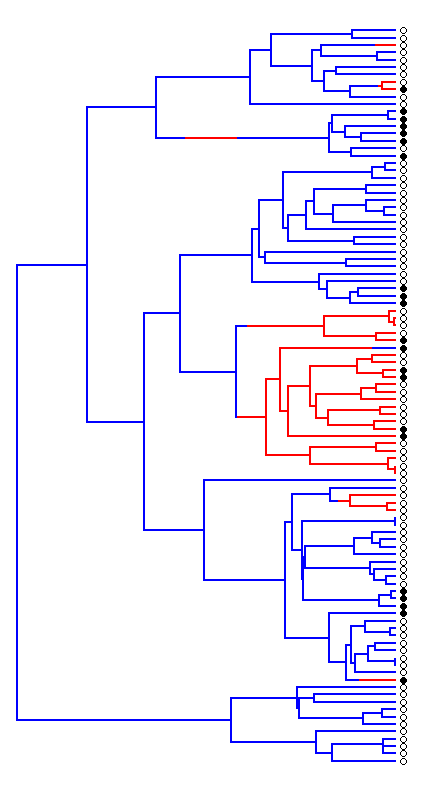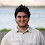## Tuesday, December 5, 2017

### Fitting a variable-process model of discrete character evolution on the tree using phytools

Now for something a little different.

Today, I have built a new method that fits a model of discrete character evolution in which the transition matrix Q varies among different parts of the tree.

These might be edges of clades specified arbitrarily by the user (for instance, using paintBranches or paintSubTree in phytools), or they could be regimes mapped onto the tree using the procedure of stochastic character mapping.

The way I did this was pretty simple. I just took the function that implements Felsenstein's famous pruning algorithm to compute the likelihood, but then I modified so that it could use a different Q for different edges. The only complication was that we might like our regime to change along an edge rather than merely at a node. To solve that, I used the phytools function map.to.singleton to convert our "simmap" object with singleton nodes and only a single regime per edge. Problem solved.

Note that this function, like fitMk, uses code for the pruning algorithm adapted from Emmanuel Paradis' ape package.

Let's try it.

First, our data:

library(phytools)
packageVersion("phytools")

##  '0.6.50'

plot(tree,ftype="off",colors=setNames(c("blue","red"),
mapped.states(tree)),xlim=c(0,1.05*max(nodeHeights(tree))))
tiplabels(pie=to.matrix(x,c(0,1)),piecol=c("black","white"),
cex=0.4,offset=0.01)So the idea is simply that we will fit a model in which the rate of transition between 0 & 1 (show here as black & white) depends on the state (red or blue) mapped onto the edges of the tree. Right?

Let's fit our model:

fitERmulti<-fitmultiMk(tree,x,model="ER")
fitERmulti

## Object of class "fitmultiMk".
##
## Fitted value of Q[a]:
##           0         1
## 0 -0.646581  0.646581
## 1  0.646581 -0.646581
##
## Fitted value of Q[b]:
##           0         1
## 0 -10.14495  10.14495
## 1  10.14495 -10.14495
##
## Fitted (or set) value of pi:
##   0   1
## 0.5 0.5
##
## Log-likelihood: -35.883658
##
## Optimization method used was "nlminb"


Or an "ARD" model that differs between parts of the tree:

fitARDmulti<-fitmultiMk(tree,x,model="ARD")
fitARDmulti

## Object of class "fitmultiMk".
##
## Fitted value of Q[a]:
##           0         1
## 0 -2.218063  2.218063
## 1  0.604146 -0.604146
##
## Fitted value of Q[b]:
##           0         1
## 0 -25.36092  25.36092
## 1  12.73165 -12.73165
##
## Fitted (or set) value of pi:
##   0   1
## 0.5 0.5
##
## Log-likelihood: -34.515745
##
## Optimization method used was "nlminb"


Of course, we can compare this, if we'd like, to a model with but a single regime on the tree. For instance:

fitER<-fitMk(tree,x,model="ER")
fitER

## Object of class "fitMk".
##
## Fitted (or set) value of Q:
##           0         1
## 0 -1.651121  1.651121
## 1  1.651121 -1.651121
##
## Fitted (or set) value of pi:
##   0   1
## 0.5 0.5
##
## Log-likelihood: -41.613061
##
## Optimization method used was "nlminb"


This suggests that our data justifies the greater model complexity of multiple regimes on the tree. That's good, because we simulated them that way!

tree<-pbtree(n=100,tip.label=LETTERS,scale=0.5)
Q<-matrix(c(-1,1,1,-1),2,2)
rownames(Q)<-colnames(Q)<-letters[1:2]
tree<-sim.history(tree,Q,anc="a")
sim.tree<-as.phylo(tree)
q<-setNames(c(1,10),letters[1:2])
sim.tree$edge.length<-colSums(t(tree$mapped.edge[,letters[1:2]])*q)
rownames(Q)<-colnames(Q)<-0:1
x<-as.factor(sim.history(sim.tree,Q)\$states)


(As of yet there is not function to simulate multiple Mk models in different parts of the tree - so what I did above is to stretch the edge lengths of the tree by regime, and simulate under a constant regime on the stretched tree.)

1.This seems like an overly complicated solution. Why not just make a covarion-like model? That is to say, you embed different 2 X 2 rate matrices into a (2 X N) X (2 X N) rate matrix, where N is the number of "regimes." The 2 X 2 rate matrices go along the diagonal. Along the off diagonal 2 X 2 areas, you have a rate of switching from one regime to another. As an aside, this is not new at all. It's an extension of the covariant model. My colleagues and I did something identical, though computationally more intensive, for selection regimes: we embedded three codon models into a 183 X 183 rate matrix, allowing switching among selection regimes.

Guindon, S., A. G. Rodrigo, K. A. Dyer, and J. P. Huelsenbeck. 2004. Modeling the site-specific variation of selection patterns along lineages. {\it Proceedings of the National Academy of Sciences, U.S.A.} 101(35):12957--12962.

1.Hi John.

I believe this is what Beaulieu et al. (2013) published, and they specifically point out that it is a generalization of the covarion model.

Please correct me if I'm wrong, of course, but this is not the same, so far as I can tell - in the sense that the regimes we are computing the likelihood over are fixed. That means, for instance, that we might have one time period (from t=0 to t=0.25, say) on the tree that we permit to evolve under one process, than a second under a different process, and so on. That is - the regime 'paintings' are observed or specifically hypothesized a priori. By contrast in the covarion model & its guild we have an unobserved set of regimes & we compute the likelihood by integrating over the probability that each datum comes from each regime.

Note that this is not better or more sophisticated than the covarion or 'hidden-rates' model - in fact, it is less! However, it does suit a specific class of hypothesis that is common in phylogenetic comparative biology - for instance that trait evolution differs between clades or among geological eras, and allows us to contrast that hypothesis against one in which it does not differ or varies in a different way.

Thanks for pointing out the relationship to the covarion model. This is a very important literature, of course!

- Liam

2.Beaulieu et al.'s corhmm is an extension of the covarion model that does what John suggests. It does rate reconstruction and ancestral state estimates at nodes (and of course it's then straightforward do make a stochastic character mapping version).

It's an interesting comparison of Liam's approach vs corhmm. Liam's requires mapping a regime on the tree, and having the rate matrix change based on the regime mapping. Corhmm doesn't require the mapping ahead of time, in effect allowing it to be inferred (the placement of the hidden state). It's like Brownie (or brownie.lite) and OUwie requiring pre-mapped regimes rather than auteur and SURFACE inferring the regime shifts [for standardized testing fans, Liam new method is to corHMM as OUwie is to SURFACE]. I can see biological use cases for both the pre-mapped regime case and the hidden regime case.

3.Incidentally (and this is a cool feature added by Jeremy Beaulieu, not me), the rayDISC function in corHMM is one of the coolest unknown tools in phylogenetics to me. Once you convert multiple characters (say, three binary traits) into a single multistate character (000 = state 0, ..., 111 = state 7) you can create very flexible rate matrices. So you can say "I can go from 000 to 001 at the same rate as 000 to 010, but 000 -> 100 is forbidden, and...."). I bring it up here because if you had a case where you thought one trait affected evolution of another, rather than stochastically mapping one and then using that to fit regimes, you probably get a better fit by inferring the mapping and the rates jointly (so you have a matrix that has the 0->1 rate for trait 1 depending on the state of trait 2, etc.). [see https://academic.oup.com/sysbio/article/62/2/339/1668230 for why the mapping one trait then estimating rate of the other might be less good than doing it jointly. ;-)]

4.To add to your discussion. I used corHMM's raydisk function to do an extension of the covarion model with very large Q-matrices. You can do LRT there and not only type I error but full power analyses. The pruning algorithm in corHMM is really efficient allowing you to work with large phylogenies. What I added to raydisk was an efficient yet decently accurate way to calculate exp{Qt}. I know phytools uses expm from package matrix but in the case of large and sparse Q matrices (>20) is nor efficient nor accurate.

Note: due to the very large amount of spam, all comments are now automatically submitted for moderation.